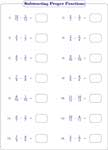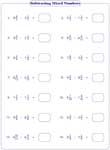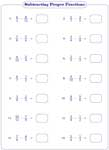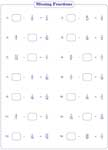# Fraction Subtraction Worksheets

Fraction subtraction practice worksheets on subtracting like and unlike fractions; subtracting special fractions; finding missing fractions and more are available for kids from 3rd through 6th grade. They also include subtracting proper, improper and mixed fractions. Range of denominators varies from 2 to 12 in easy level; 2 to 20 in moderate level and 2 to 99 in difficult level.

## Fraction Subtraction using Number Lines

This page features 50+ practice worksheets focusing on the concept of fraction subtraction on a number line.Subtracting Fractions using a Number Line Model (60 Worksheets)

## Like Fractions - Same Denominators

Subtracting proper fractions

These worksheets are on subtracting proper fractions with the same (like) denominators. The worksheets are categorized into easy, moderate and difficult levels.Easy (Horizontal):

Easy (Vertical):

Moderate (Horizontal):

Moderate (Vertical):

Difficult (Horizontal):

Subtracting improper fractions

Practice subtracting improper fractions in both horizontal and vertical forms in these worksheets.Easy (Horizontal):

Easy (Vertical):

Moderate (Horizontal):

Moderate (Vertical):

Difficult (Horizontal):

Subtracting mixed numbers

Convert the mixed numbers to fractions and preform the subtraction operation in these worksheets. Ample practice sheets are here for children.Easy (Horizontal):

Easy (Vertical):

Moderate (Horizontal):

Moderate (Vertical):

Difficult (Horizontal):

Subtracting like fractions: Mixed review

The worksheets have proper, improper and mixed fractions. Subtract the pairs of fractions given in different combinations.Proper / Improper:

Proper / Mixed:

Improper / Mixed:

All fractions:

## Unlike Fractions - Different Denominators

Subtracting proper fractions

The students should subtract each pair of fractions with unlike denominators. They should have good knowledge in least common multiple to find the solution. Requires simplification.Easy (Horizontal):

Easy (Vertical):

Moderate (Horizontal):

Moderate (Vertical):

Difficult (Horizontal):

Subtracting improper fractions

Subtract the improper fractions and reduce them to the simplest form. The denominators are different.Easy (Horizontal):

Easy (Vertical):

Moderate (Horizontal):

Moderate (Vertical):

Difficult (Horizontal):

Subtracting mixed numbers

Find the solution by subtracting the mixed fractions with different denominators. Row and column subtractions are included.Easy (Horizontal):

Easy (Vertical):

Moderate (Horizontal):

Moderate (Vertical):

Difficult (Horizontal):

Subtracting unlike fractions: Mixed review

Boost up your knowledge by practicing these worksheets on subtracting unlike fractions. Select the range of fractions you want to explore.Proper / Improper:

Proper / Mixed:

Improper / Mixed:

All fractions:

## Subtracting Special Fractions

Subtracting fractions from the whole

Each worksheet contains 14 problems on subtracting proper, improper and mixed fractions from whole numbers. A mixed review of fraction subtraction problems is also included.Proper:

Improper:

Mixed Numbers:

Mixed Review:

Unit Fractions

Unit fractions have one in the numerator and positive integer in the denominator. Subtract the unit fractions given in these worksheets.Proper:

Mixed Numbers:

Mixed Review:

## Missing Fractions

Same denominators

Focus on the numerators and identify the missing fraction in each problem. The fractions are in various forms: proper, improper and mixed.Proper:

Improper:

Mixed Numbers:

All fractions:

Different denominators

In each problem, move the fraction from the left to the right and add them to solve the problem. Write the answer in the simplest form.Proper:

Improper:

Mixed Numbers:

All fractions:

Finding value of variables

Find the value of the variable in each problem. The worksheets have either like fractions or unlike fractions.Same Denominators:

Different Denominators:

Stock up your library with the complete set of worksheets on subtracting fractions.

Related Worksheets